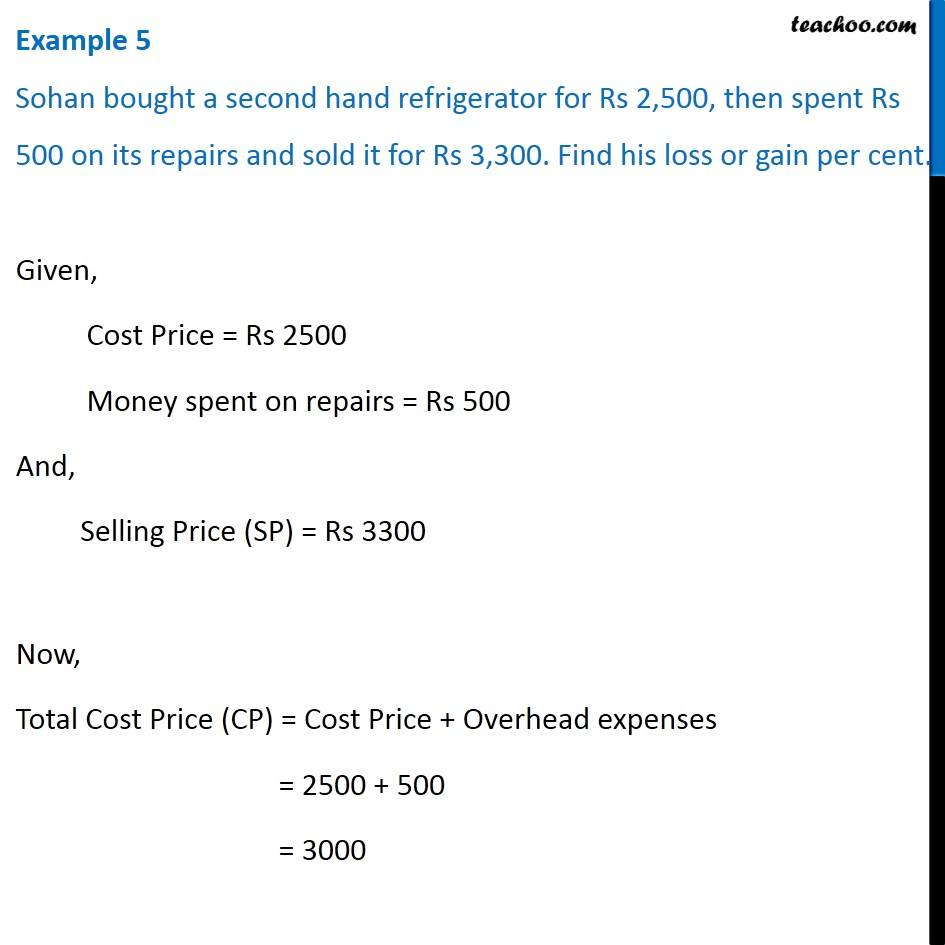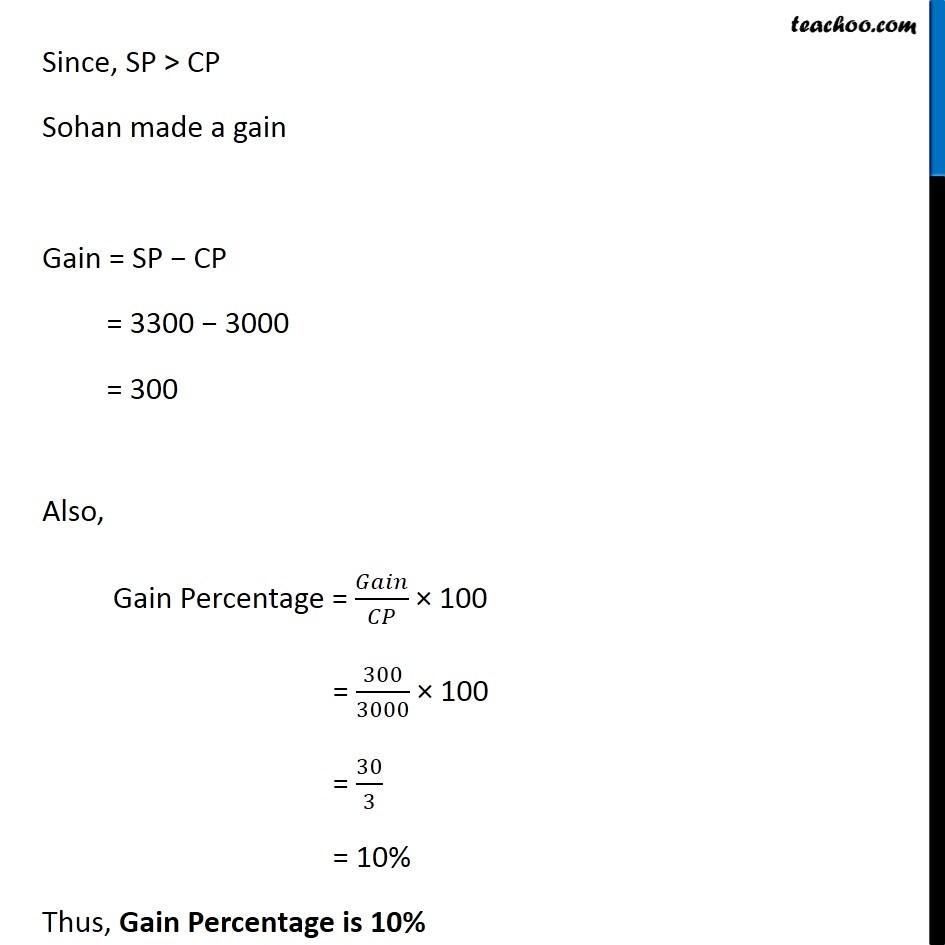Examples

Chapter 7 Class 8 Comparing Quantities
Serial order wiseLearn in your speed, with individual attention - Teachoo Maths 1-on-1 Class

### Transcript

Question 2 Sohan bought a second hand refrigerator for Rs 2,500, then spent Rs 500 on its repairs and sold it for Rs 3,300. Find his loss or gain per cent. Given, Cost Price = Rs 2500 Money spent on repairs = Rs 500 And, Selling Price (SP) = Rs 3300 Now, Total Cost Price (CP) = Cost Price + Overhead expenses = 2500 + 500 = 3000 Since, SP > CP Sohan made a gain Gain = SP − CP = 3300 − 3000 = 300 Also, Gain Percentage = 𝐺𝑎𝑖𝑛/𝐶𝑃 × 100 = 300/3000 × 100 = 30/3 = 10% Thus, Gain Percentage is 10%